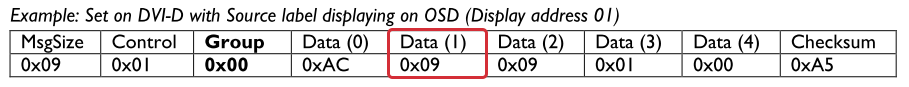# Checksum calculation in level sequences

For calculating checksum in level sequences you will need to make a dynamic calculating string.

In the example below we will use the Philips protocol!

Always start by reading the protocol of the device thoroughly.
All good configuration starts this way!
In section 2.3 of the Philips RS232 protocol we see the structure for the messages we are about to construct for the Philips display.

Notice the way the Checksum is calculated, in this case it’s done with an XOR function.Philips uses a protocol, where the ensure the integrity of the sent message by calculating the checksum!

The checksum is a mathematical calculation of all of the bytes prior to the checksum!You need Biamp Device Editor and Windows calculator in programme mode in order to calculate the checksum:In this case we would like to change an input, therfore we have to do the following:

• Find the right section in the protocol(Message Set)
• Choose the input we want to set (HDMI 2)
• Note the command and use the example in order to edit our command.Rewrite the command so that it fits:

Take the this:And this:Now what is the checksum?:Checksum

Now add all of the bytes like this:09 Xor 01 Xor 00 Xor AC Xor 06 Xor 09 Xor 01 Xor 00 = Checksum = AAPhilips uses the Xor function, but normal addition is also very common in other protocols.

Now insert the string into Biamp Device Editor:This command is done, and the procedure can be repeated for all other controls:The next thing is to create a step volume control, where the checksum is calculated automatically.

Find the correct values in the protocol:Insert them in Device Editor:______________________________________________________________________________

Now learn your device driver to calculate the checksum automatically.

Select the bytes you wish to calculate:Select the target byte, where to put the checksum calculation:Choose the method of calculation:Define the field where the count is being performed.

Place your cursor in the field:Field is marked:Now press "Count" and "Define first count":The field now turns purple:Now Choose the in/decrease value, repeat time and step value:The procedure is the same for LAN!

Please also find this description attached as PDF.